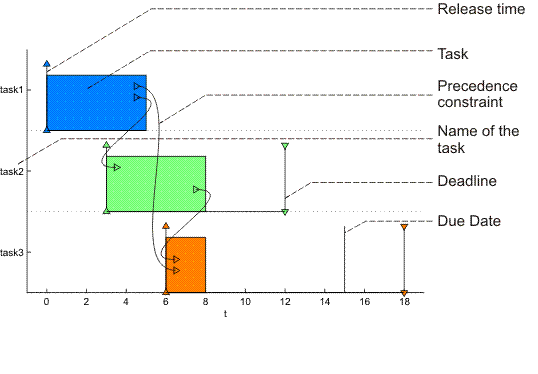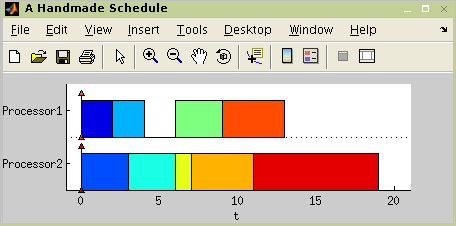"; } ?> Sets of Tasks

# TORSCHE Scheduling Toolbox for Matlab

## TORSCHE – Sets of Tasks

### 1. Creating the `taskset` Object

Objects of the type task can be grouped into a set of tasks. A set of tasks is an object of the type `taskset` which can be created by the command `taskset`. Syntax for this command is:

`T = taskset(tasks[,prec])`

where variable `tasks` is an array of objects of the type `task`. Furthermore, relations between tasks can be defined by precedence constraints in parameter `prec`. Parameter `prec` is an adjacency matrix (see Chapter 6, Graphs) defining a graph where nodes correspond to tasks and edges are precedence constraints between these tasks. If there is an edge from `T`i to `T`j in the graph, it means that `T`i must be completed before `T`j can be started.

If there are not precedence constraints between the tasks, we can use a shorter form of creating a set of tasks using square brackets (see the first line in Figure 4.1, “Creating a set of tasks and adding precedence constraints”).

```>> T1 = [t1 t2 t3]

>> T1 = taskset(T1,[0 1 1; 0 0 1; 0 0 0])
There are precedence constraints

>> T2 = taskset([3 4 2 4 4 2 5 4 8])

You can also create a set of tasks directly from a vector of processing times. Call the command `taskset` as shown in Figure 4.1, “Creating a set of tasks and adding precedence constraints”. Tasks with those processing times will be automatically created inside the set of tasks. Precedence constraints can be added in the same way as in case of taskset `T1` (see Figure 4.1, “Creating a set of tasks and adding precedence constraints”).

### 2. Graphical Representation of the Set of Tasks

As for single tasks, command `plot` can be used to draw parameters of set of tasks graphically. An example of plot output with explanation of used marks is shown in Figure 4.2, “Gantt chart for a set of scheduled tasks”. For more details see Reference Guide @taskset/plot.m.

`>> plot(T1)`Figure 4.2. Gantt chart for a set of scheduled tasks

### 3. Set of Tasks Modification

Commands changing parameters of tasksets are the same as for task object. Command `get` returns the value of the specified property or values of all properties. Command `set` sets the value of the specified property. These two commands has got a standard syntax, which is described in Matlab user manual. Property access is allowed over the . (dot) operator too.

Note

To obtain a list of all accessible properties use command `get`. Note that some private and virtual properties aren't accessible over the . (dot) operator, although they are displayed when the automatic completion by Tab key is used.

Tasks parameters may be modified via virtual properties of object taskset. The list of virtual properties are: `Name`, `ProcTime`, `ReleaseTime`, `Deadline`, `DueDate`, `Weight`, `Processor`, `UserParam`. All parameters are arrays data type. Items order in the arrays is the same as tasks order in the set of the tasks.

```>> T2.ProcTime
ans =
3     4     2     4     4     2     5     4     8
>> T2.ProcTime(3) = 5;
>> T2.ProcTime
ans =
3     4     5     4     4     2     5     4     8
>> T2.ProcTime = T2.ProcTime -1;
>> T2.ProcTime
ans =
2     3     4     3     3     1     4     3     7  ```

#### 3.2. Schedule

The only way how to operate with schedule of tasks is through commands `add_schedule` and `get_schedule`. Command `add_schedule` inserts a schedule (i.e. start time `s`j, number of assigned processor, ...) into taskset object. Its syntax is described in Reference Guide @taskset/add_schedule.m. An example of `add_schedule` command use is shown in Figure 4.4, “Schedule inserting example”. Vector `start` is vector of start times (i.e. first task starts at 0), vector processor is vector of assigned processors (i.e. first task is assigned to the firs processor) and string description is a brief note on used scheduling algorithm.

```>> start = [0 0 2 3 6 6 7 9 11];
>> processor = [1 2 1 2 1 2 2 1 2];
>> description = 'a handmade schedule';
>>
>> get_schedule(T2)
ans =
0     0     2     3     6     6     7     9    11
>> plot(T2);```Figure 4.4. Schedule inserting example

On the other hand, the schedule can be obtained from a taskset using command `get_schedule` (e.g. as is shown in Figure 4.4, “Schedule inserting example”). For more details about this function see Reference Guide @taskset/get_schedule.m. Graphical schedule interpretation (Gantt chart) can be obtained using function `plot`.

Parameters of a given schedule (e.g. value of optimality criteria, solving time, ...) can be obtained using function `schparam`. It returns information about schedule inside the taskset and its syntax is described in Reference Guide @taskset/schparam.m. An example of use is shown in Figure 4.5, “Schedule parameters”.

```>> param = schparam(T2,'cmax')
param =
19

>> param = schparam(T2)
param =
cmax: 19
sumcj: 80
sumwcj: 80```

Figure 4.5. Schedule parameters

### 4. Other Functions

#### 4.1. Count and Size

Commands `count(T)` and `size(T)` return number of tasks in the set of tasks `T`. At this moment they return the same value. Returned value will be different after implementing the general shop problems into the toolbox. Now it is recommended to use command `count`.

#### 4.2. Sort

The function returns sorted set of tasks inside taskset over selected parameter. Its syntax is described in Reference Guide @taskset/sort.m. An example is shown in Figure 4.6, “Taskset sort example”.

```>> T2.ProcTime
ans =
2     3     4     3     3     1     4     3     7
>> T3 = sort(T2,'ProcTime','dec');
>> T3.ProcTime
ans =
7     4     4     3     3     3     3     2     1```

Random taskset `T` can be created by the command `randtaskset`. Tasks parameters in the taskset are generated with a uniform distribution. The syntax is described in Reference Guide randtaskset.m. Example of its application is shown in Figure 4.7, “Example of random taskset use”.

```>> T = randtaskset(8,[8 15],[3 6]);

>> T.ProcTime
ans =
15    12    14    11    14    12    14     9

>> T.ReleaseTime
ans =
4     4     5     3     4     5     5     4```

Figure 4.7. Example of random taskset use

Note

Random task can be created by command `randtask`.

Webmaster - Jan Dvořák (2004 - 2020)